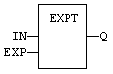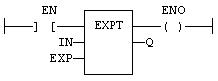# EXPT

Function - Calculates a power.

## Inputs

IN : REAL   Real value
EXP : DINT  Exponent

## Outputs

Q : REAL   Result: IN at the 'EXP' power

## Remarks

In LD language, the operation is executed only if the input rung (EN) is TRUE. The output rung (ENO) keeps the same value as the input rung. In IL, the input must be loaded in the current result before calling the function. The exponent (second input of the function) must be the operand of the function.

## ST Language

Q := EXPT (IN, EXP);

## FBD Language## LD Language

(* The function is executed only if EN is TRUE *)
(* ENO keeps the same value as EN *)## IL Language:

Op1: LD   IN
EXPT EXP
ST   Q    (* Q is: (IN ** EXP) *)